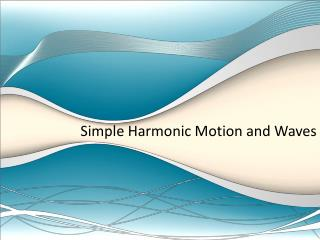# Simple Harmonic Motion and Waves - PowerPoint PPT PresentationDownload PresentationSimple Harmonic Motion and Waves

Simple Harmonic Motion and WavesDownload Presentation## Simple Harmonic Motion and Waves

- - - - - - - - - - - - - - - - - - - - - - - - - - - E N D - - - - - - - - - - - - - - - - - - - - - - - - - - -
##### Presentation Transcript

1. Simple Harmonic Motion and Waves

2. Simple Harmonic Motion • Simple harmonic motion (SHM) refers to any object that oscillates back and forth. • A pendulum exhibits SHM • As you learned in the lab

3. Pendulums • A pendulum is defined as any mass suspended from a fixed point and allowed to swing freely back and forth • Period – amount of time required to complete one oscillation (cycle). • Factors that effect the period of a pendulum: • Length of pendulum • Acceleration due to gravity

4. Waves • Waves are like objects in SHM because the particles of the medium oscillate back and forth. • Medium – the material through which a wave propagates (moves)

5. Waves • Wave – a rhythmic disturbance that carries energy through a medium • WAVES TRANSMIT ENERGY • There are two categories of waves you need to be familiar with • Mechanical waves • Electromagnetic waves

6. Electromagnetic waves • Consist of alternating electric and magnetic fields • Do NOT require a physical medium (they can travel through empty space) • We will discuss electromagnetic waves in more detail when we discuss light.

7. Mechanical waves • Mechanical waves are waves that MUST travel through a medium. They cannot travel through empty space. • Two types of mechanical waves • Longitudinal (compression) waves • Transverse waves

8. Longitudinal Waves • These are waves in which the particles of the medium oscillate (vibrate) parallel to the direction of wave propagation (motion)

9. Transverse Waves • These are waves in which the particles of the medium oscillate (vibrate) perpendicular to the direction of wave propagation (motion)

10. Words Associated with Waves • Medium – material the wave travels through • Wavelength – length of one wave • Measured in meters • Symbol is the Greek letter lambda (l) • Frequency – the number of complete oscillations (cycles) per second • Measured in reciprocal seconds (s-1) also known as Hertz (Hz) • Symbol is a lowercase f.

11. Words associated with Waves • Amplitude – the maximum displacement of the particles of the medium from their resting point • Shows the amount of energy being transmitted • Crest – point of greatest positive displacement on a transverse wave • Trough – point of greatest negative displacement on a transverse wave

12. Words associated with waves • Compression – point of greatest pressure in a longitudinal wave • Rarefaction – point of least pressure in a longitudinal wave • Wave speed – how fast the wave is travelling • Wave speed depends only on the medium through which it travels.

13. Anatomy of a Transverse Wave

14. Anatomy of a Longitudinal Wave

15. Wave Speed Equation • Speed – v – in meters per second • Wavelength – λ– in meters • Frequency – f – in s-1 • So… v = λ∙f

16. The End Of day 1

17. A sound wave produced by a clock chime is heard 515m away 1.50s later. What is the speed of sound of the clock’s chime in air? If the wave has a frequency of 436 Hz. What is its period? What is its wavelength? f = 436 Hz d = 515 m f = 436 Hz v = 343 m/s t = 1.50 s T = 1 / f v = λ∙f v = d / t T = 1/436 Hz T = 0.00229 s λ= v / f v = 515 m / 1.50 s λ= (343 m/s) / (436 Hz) v = 343 m/s λ = 0.787 m

18. Wave Behavior Mechanical wave speed depends on the medium it is traveling through. -Air temperature -Depth of liquid -Mass of spring/rope

19. Boundaries When a wave hits a boundary some energy is transferred to the new medium & some is reflected. Incident wave – a wave that strikes & carries through a boundary. Reflected wave – wave that is reflected (bounces back).

20. Boundaries Boundary types – soft = movable - fixed = stationary (not moving) Reflected waves: soft boundary = upward reflection fixed boundary = inverted reflection

21. Superposition Principle of Superposition– 2 or more waves combing to form a new wave. Interference – the result of superposition.

22. Interference Destructive Interference– equal & opposite amplitudes. -Displacement is zero.

23. Interference Constructive Interference – wave displacements in the same direction. -Displacement is the sum of the 2 individual waves.

24. Interference Unequal Destructive Interference – wave intersecting that are opposite & unequal. Resultant is the sum of the waves. (like finding net force)

25. Wave Behavior Reflection – 2 dimensional waves reflect at different angles when striking a barrier. Law of Reflection – the angle of incidence equals the angle of reflection. Normal Angle of Incidence Angle of reflection

26. Wave Behavior Refraction – change in direction of waves at a boundary between 2 mediums. Frequency will not change, however wavelength can change between mediums.

27. Wave Behavior Diffraction – spreading of waves around a barrier. Waves will expand after intersection with a barrier & interfere with each other. Wave traveling through a hole will form circular waves on the other side of the barrier. Smaller wavelength = less diffraction.

28. Wave Behavior Waves will expand after intersection with a barrier & interfere with each other.

29. E D